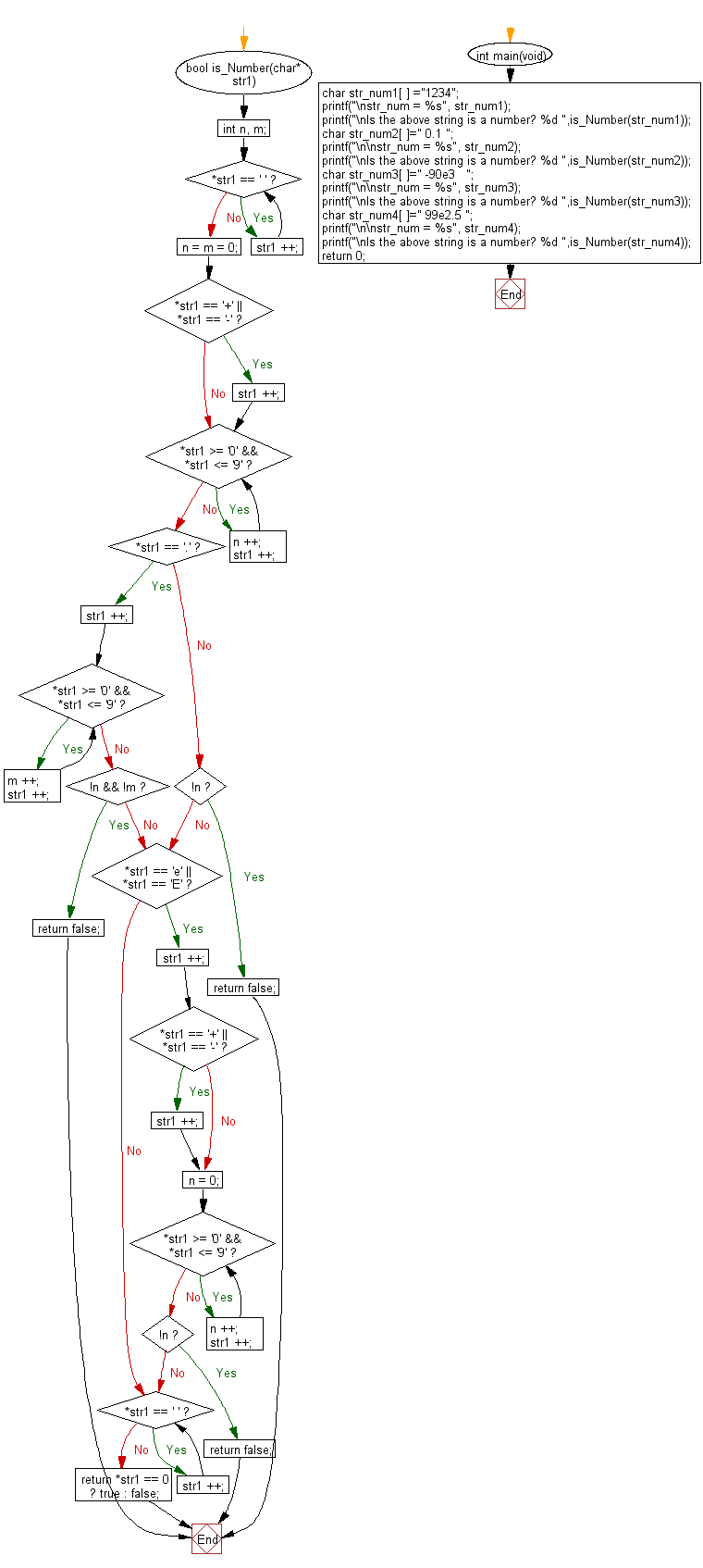﻿ C Program: If a string can be interpreted as a decimal

# C Exercises: Check if a given string can be interpreted as a decimal number

## C Programming Mathematics: Exercise-6 with Solution

Write a C program to check if a given string can be interpreted as a decimal number.

Example:
Input:
str_num1[ ] ="1234"
str_num2[ ]=" 0.1 "
str_num3[ ]=" -90e3 "
str_num4[ ]=" 99e2.5 "
Output:
Is the above string is a number? 1
Is the above string is a number? 1
Is the above string is a number? 1
Is the above string is a number? 0

Sample Solution:

C Code:

``````#include <stdio.h>
#include <stdlib.h>
#include <string.h>
#include <stdbool.h>

bool is_Number(char* str1) {
int n, m;

while (*str1 == ' ') str1 ++;

n = m = 0;

// skip the sign of the number
if (*str1 == '+' || *str1 == '-') str1 ++;

while (*str1 >= '0' && *str1 <= '9') {
n ++;
str1 ++;
}

if (*str1 == '.') {
str1 ++;
while (*str1 >= '0' && *str1 <= '9') {
m ++;
str1 ++;
}
if (!n && !m) return false;
} else if (!n) {
return false;
}

if (*str1 == 'e' || *str1 == 'E') {
str1 ++;
if (*str1 == '+' || *str1 == '-') str1 ++;
n = 0;
while (*str1 >= '0' && *str1 <= '9') {
n ++;
str1 ++;
}
if (!n) return false;
}

while (*str1 == ' ') str1 ++;

return *str1 == 0 ? true : false;
}

int main(void)
{
char str_num1[ ] ="1234";
printf("\nstr_num = %s", str_num1);
printf("\nIs the above string is a number? %d ",is_Number(str_num1));
char str_num2[ ]=" 0.1 ";
printf("\n\nstr_num = %s", str_num2);
printf("\nIs the above string is a number? %d ",is_Number(str_num2));
char str_num3[ ]=" -90e3   ";
printf("\n\nstr_num = %s", str_num3);
printf("\nIs the above string is a number? %d ",is_Number(str_num3));
char str_num4[ ]=" 99e2.5 ";
printf("\n\nstr_num = %s", str_num4);
printf("\nIs the above string is a number? %d ",is_Number(str_num4));
return 0;
}
```
```

Sample Output:

```str_num = 1234
Is the above string is a number? 1

str_num =  0.1
Is the above string is a number? 1

str_num =  -90e3
Is the above string is a number? 1

str_num =  99e2.5
Is the above string is a number? 0
```

Flowchart:C Programming Code Editor:

Improve this sample solution and post your code through Disqus.

What is the difficulty level of this exercise?

Test your Programming skills with w3resource's quiz.

﻿